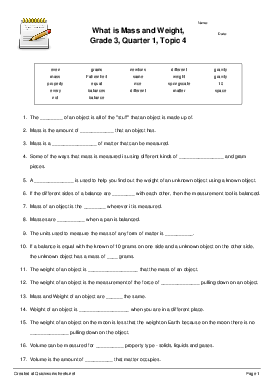# What is Mass and Weight, Grade 3, Quarter 1, Topic 4

Fill-in-the-Blank Sentences Worksheet

Kindly Shared By:United States of America

Date Shared: 12 October 2016

Worksheet Type:

Tags Describing Content or Audience:

Worksheet Instructions:

None provided.Target Language:

The mass of an object is all of the "stuff" that an object is made up of. Mass is the amount of matter that an object has. Mass is a property of matter that can be measured. Some of the ways that mass is measured is using different kinds of balances and gram pieces. A balance is used to help you find out the weight of an unknown object using a known object. If the different sides of a balance are even with each other, then the measurement tool is balanced. Mass of an object is the same wherever it is measured. Masses are equal when a pan is balanced. The units used to measure the mass of any form of matter is grams. If a balance is equal with the known of 10 grams on one side and a unknown object on the other side, the unknown object has a mass of 10 grams. The weight of an object is different that the mass of an object. The weight of an object is the measurement of the force of gravity pulling down on an object. Mass and Weight of an object are not the same. Weight of an object is different when you are in a different place. The weight of an object on the moon is less that the weight on Earth because on the moon there is no gravity pulling down on the object. Volume can be measured for every property type - solids, liquids and gases. Volume is the amount of space that matter occupies. On every planet, the weight of matter/object is different because the force of gravity is different on every planet. A springscale is used to measure the weight of an object. The greater the force of gravity, the more that the spring is pushed down. The metric units used by scientist to measure the weight of an object is newtons. Temperature is measured by two different unit systems - Fahrenheit and Celsius. The celsius rhyme says thirty is hot, twenty is nice, ten is cool and zero is ice.

1) What are the four different kinds of balances? 2) What is temperature?12 October 2016

#### cabutler017AuthorUnited States of America

More on Mass, Weight for 3rd Grade Science